# Backtracking Sum of Subsets and Knapsack Backtracking Two

• Slides: 42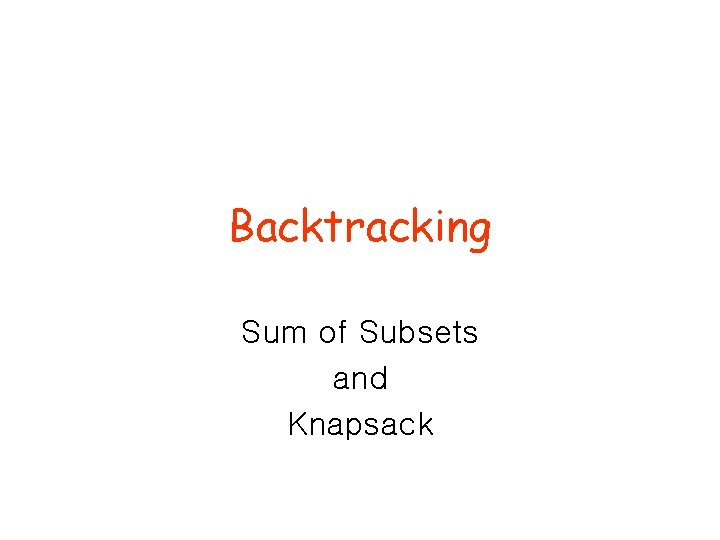Backtracking Sum of Subsets and KnapsackBacktracking • Two versions of backtracking algorithms – Solution only needs to be feasible (satisfy problem’s constraints) • sum of subsets – Solution needs also to be optimal • knapsackThe backtracking method • A given problem has a set of constraints and possibly an objective function • The solution must be feasible and it may optimize an objective function • We can represent the solution space for the problem using a state space tree – – The root of the tree represents 0 choice, Nodes at depth 1 represent first choice Nodes at depth 2 represent the second choice, etc. In this tree a path from a root to a leaf represents a candidate solutionSum of subsets • Problem: Given n positive integers w 1, . . . wn and a positive integer S. Find all subsets of w 1, . . . wn that sum to S. • Example: n=3, S=6, and w 1=2, w 2=4, w 3=6 • Solutions: {2, 4} and {6}Sum of subsets • We will assume a binary state space tree. • The nodes at depth 1 are for including (yes, no) item 1, the nodes at depth 2 are for item 2, etc. • The left branch includes wi, and the right branch excludes wi. • The nodes contain the sum of the weights included so far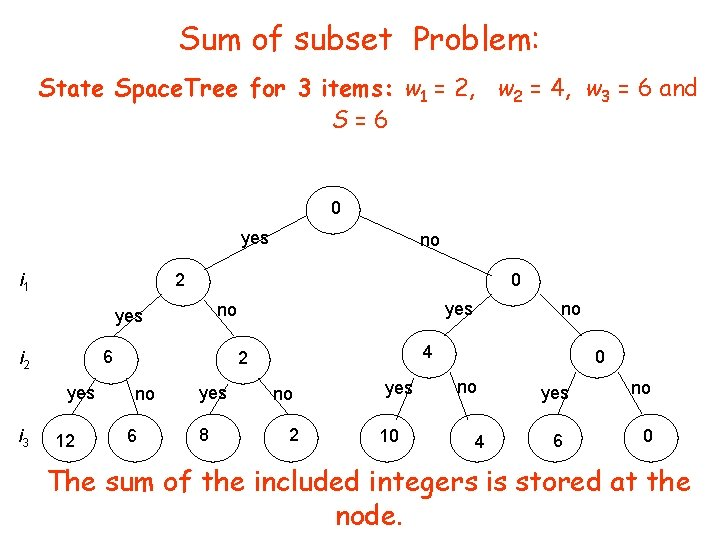Sum of subset Problem: State Space. Tree for 3 items: w 1 = 2, w 2 = 4, w 3 = 6 and S=6 0 yes i 1 2 0 6 i 2 yes 12 yes no yes i 3 no 4 2 no 6 yes 8 no no 2 yes 10 0 no 4 yes 6 no 0 The sum of the included integers is stored at the node.A Depth First Search solution • Problems can be solved using depth first search of the (implicit) state space tree. • Each node will save its depth and its (possibly partial) current solution • DFS can check whether node v is a leaf. – If it is a leaf then check if the current solution satisfies the constraints – Code can be added to find the optimal solution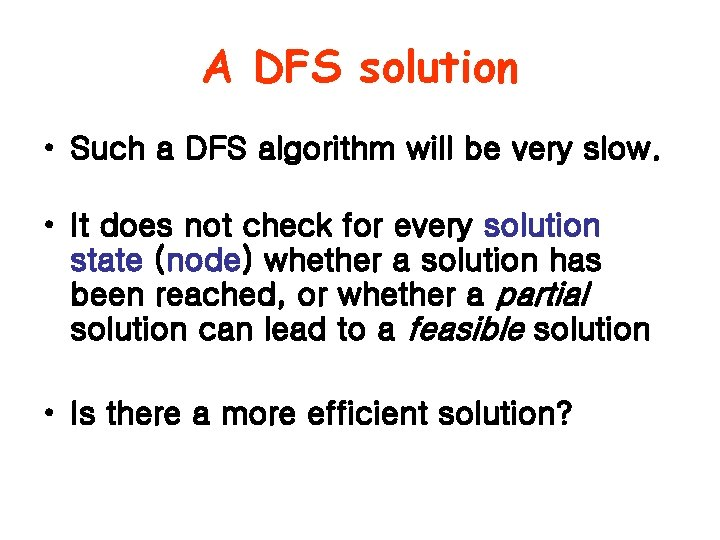A DFS solution • Such a DFS algorithm will be very slow. • It does not check for every solution state (node) whether a solution has been reached, or whether a partial solution can lead to a feasible solution • Is there a more efficient solution?Backtracking • Definition: We call a node nonpromising if it cannot lead to a feasible (or optimal) solution, otherwise it is promising • Main idea: Backtracking consists of doing a DFS of the state space tree, checking whether each node is promising and if the node is nonpromising backtracking to the node’s parentBacktracking • The state space tree consists of expanded nodes only (called the pruned state space tree) • The following slide shows the pruned state space tree for the sum of subsets example • There are only 15 nodes in the pruned state space tree • The full state space tree has 31 nodes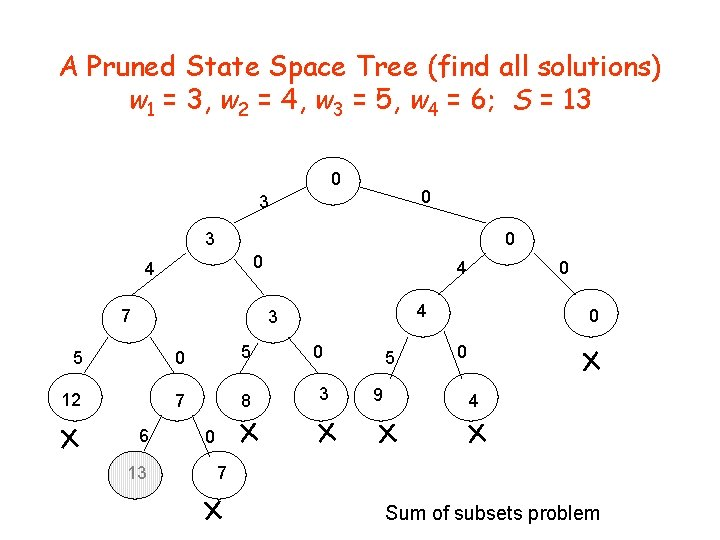A Pruned State Space Tree (find all solutions) w 1 = 3, w 2 = 4, w 3 = 5, w 4 = 6; S = 13 0 0 3 3 0 0 4 7 4 4 3 5 0 5 12 7 8 6 13 0 0 3 5 9 0 0 4 0 7 Sum of subsets problem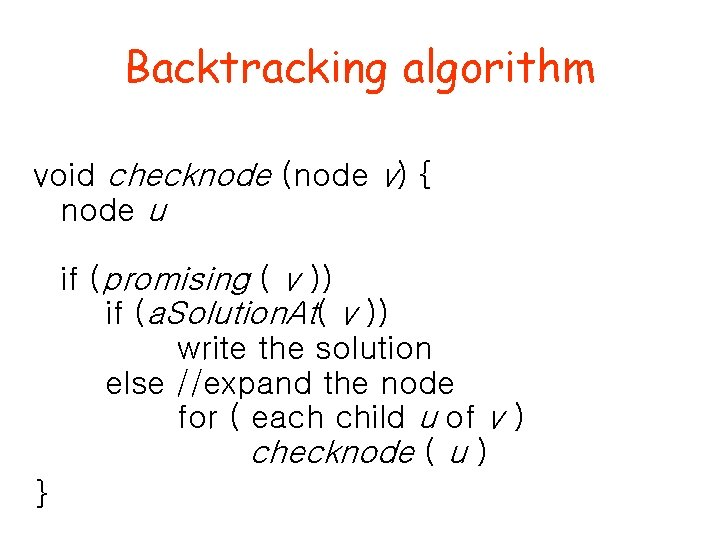Backtracking algorithm void checknode (node v) { node u if (promising ( v )) if (a. Solution. At( v )) write the solution else //expand the node for ( each child u of v ) checknode ( u ) }Checknode • Checknode uses the functions: – promising(v) which checks that the partial solution represented by v can lead to the required solution – a. Solution. At(v) which checks whether the partial solution represented by node v solves the problem.Sum of subsets – when is a node “promising”? • Consider a node at depth i • weight. So. Far = weight of a node, i. e. , sum of numbers included in partial solution that the node represents • total. Possible. Left = weight of the remaining items i+1 to n (for a node at depth i) • A node at depth i is non-promising if (weight. So. Far + total. Possible. Left < S ) or (weight. So. Far + w[i+1] > S ) • To be able to use this “promising function” the wi must be sorted in non-decreasing orderA Pruned State Space Tree w 1 = 3, w 2 = 4, w 3 = 5, w 4 = 6; S = 13 1 0 0 3 2 0 4 3 7 8 5 4 5 7 6 6 13 4 9 8 12 3 5 0 12 11 3 0 10 3 13 9 0 0 4 15 0 0 5 14 4 0 7 7 - backtrack Nodes numbered in “call” ordersum. Of. Subsets ( i, weight. So. Far, total. Possible. Left ) 1) if (promising ( i )) //may lead to solution 2) then if ( weight. So. Far == S ) 3) then print include[ 1 ] to include[ i ] //found solution return 4) else //expand the node when weight. So. Far < S 5) include [ i + 1 ] = "yes” //try including 6) sum. Of. Subsets ( i + 1, weight. So. Far + w[i + 1], total. Possible. Left - w[i + 1] ) 7) include [ i + 1 ] = "no” //try excluding 8) sum. Of. Subsets ( i + 1, weight. So. Far , total. Possible. Left - w[i + 1] ) boolean promising (i ) 1) return ( weight. So. Far + total. Possible. Left S) and ( weight. So. Far == S or weight. So. Far + w[i + 1] S ) Prints all solutions! Initial call sum. Of. Subsets(0, 0, )Backtracking for optimization problems • To deal with optimization we compute: best - value of best solution achieved so far value(v) - the value of the solution at node v – Modify promising(v) • Best is initialized to a value that is equal to a candidate solution or worse than any possible solution. • Best is updated to value(v) if the solution at v is “better” • By “better” we mean: – larger in the case of maximization and – smaller in the case of minimizationModifying promising • A node is promising when – it is feasible and can lead to a feasible solution and – “there is a chance that a better solution than the (current) best can be achieved by expanding it” • Otherwise it is nonpromising • A bound on the best solution that can be achieved by expanding the node is computed and compared to best • If the bound > best for maximization, (< best for minimization) the node is promising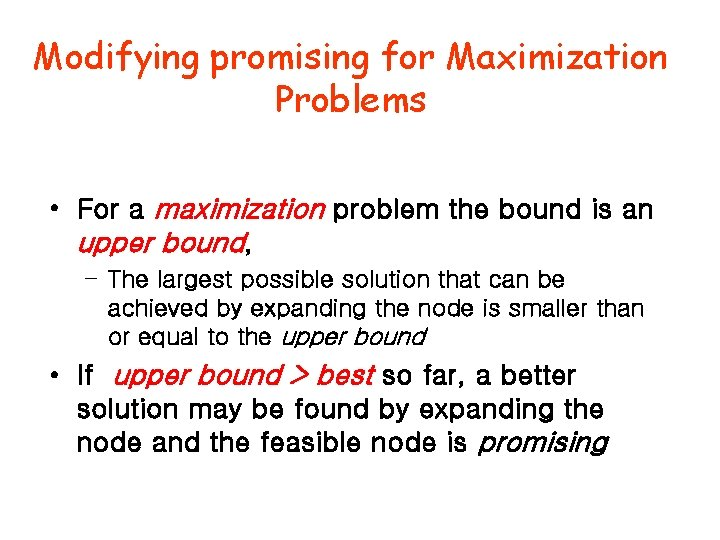Modifying promising for Maximization Problems • For a maximization problem the bound is an upper bound, – The largest possible solution that can be achieved by expanding the node is smaller than or equal to the upper bound • If upper bound > best so far, a better solution may be found by expanding the node and the feasible node is promising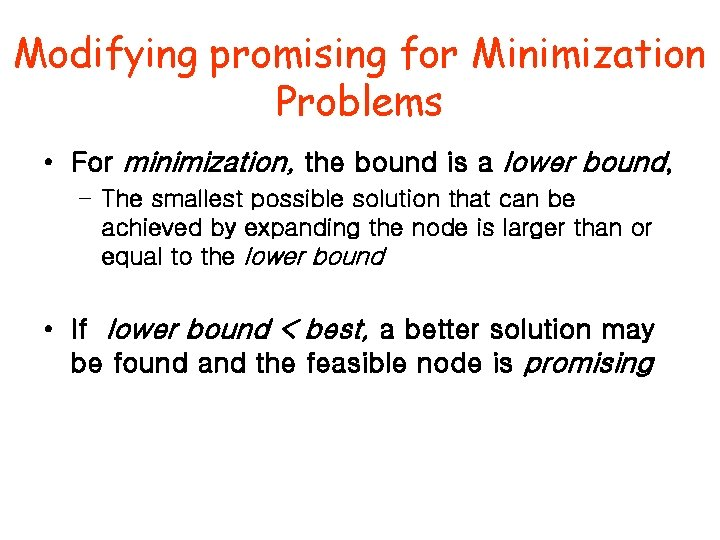Modifying promising for Minimization Problems • For minimization, the bound is a lower bound, – The smallest possible solution that can be achieved by expanding the node is larger than or equal to the lower bound • If lower bound < best, a better solution may be found and the feasible node is promising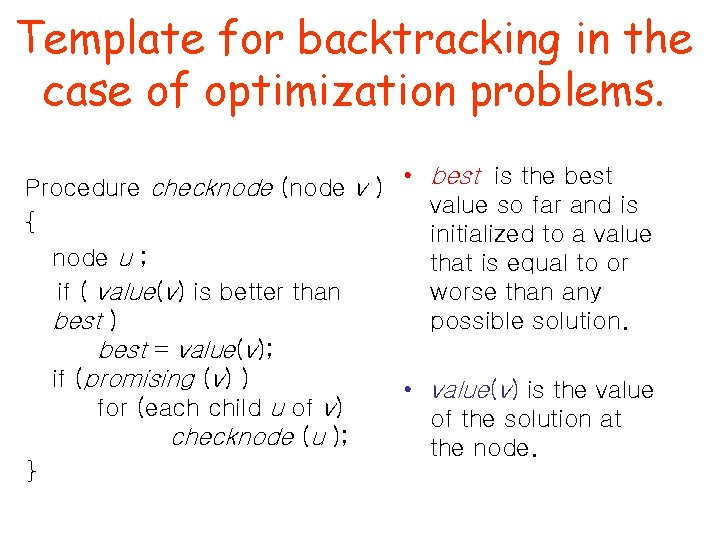Template for backtracking in the case of optimization problems. Procedure checknode (node v ) • best is the best value so far and is { initialized to a value node u ; that is equal to or worse than any if ( value(v) is better than possible solution. best ) best = value(v); if (promising (v) ) • value(v) is the value for (each child u of v) of the solution at checknode (u ); the node. }Knapsack problem • 0 -1 knapsack problem using greedy algorithm • Fractional knapsack problemNotation for knapsack • We use maxprofit to denote best • profit(v) to denote value(v)The state space tree for knapsack • Each node v will include 3 values: – profit (v) = sum of profits of all items included in the knapsack (on a path from root to v) – weight (v)= the sum of the weights of all items included in the knapsack (on a path from root to v) – upper. Bound(v) is greater or equal to the maximum benefit that can be found by expanding the whole subtree of the state space tree with root v. • The nodes are numbered in the order of expansion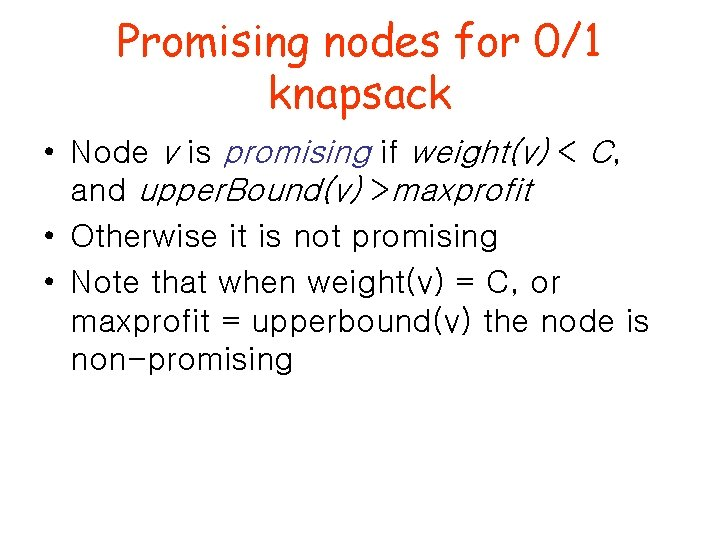Promising nodes for 0/1 knapsack • Node v is promising if weight(v) < C, and upper. Bound(v) >maxprofit • Otherwise it is not promising • Note that when weight(v) = C, or maxprofit = upperbound(v) the node is non-promisingMain idea for upper bound • Main idea: KWF (knapsack with fraction) can be used for computing the upper bounds • Theorem: The optimal profit for 0/1 knapsack optimal profit for KWF • Discussion: Clearly the optimal solution to 0/1 knapsack is a possible solution to KWF. So the optimal profit of KWF is greater or equal to that of 0/1 knapsackComputing the upper bound for 0/1 knapsack • Given node v at depth i. • Upper. Bound(v) = KWF 2(i+1, weight(v), profit(v), w, p, C, n) • KWF 2 requires that the items be ordered by non -increasing pi / wi. If we arrange the items in this order before applying the backtracking algorithm, KWF 2 will pick the remaining items in the required order.KWF 2(i, weight, profit, w, p, C, n) 1. bound = profit 2. for j=i to n 3. x[j]=0 //initialize variables to 0 4. while (weight<C && i<=n) //not “full”and more items 5. if weight+w[i]<=C //room for next item 6. x[i]=1 //item i is added to knapsack 7. weight=weight+w[i]; bound = bound +p[i]; 8. else 9. x[i]=(C-weight)/w[i] //fraction of i added to knapsack 10. weight=C; bound = bound + p[i]*x[i] 11. i=i+1 // next item 12. return bound • KWF 2 is in O(n) (assuming items sorted before applying backtracking)Pseudo code • The arrays w, p, include and bestset have size n+1. • Location 0 is not used • include contains the current solution • bestset the best solution so far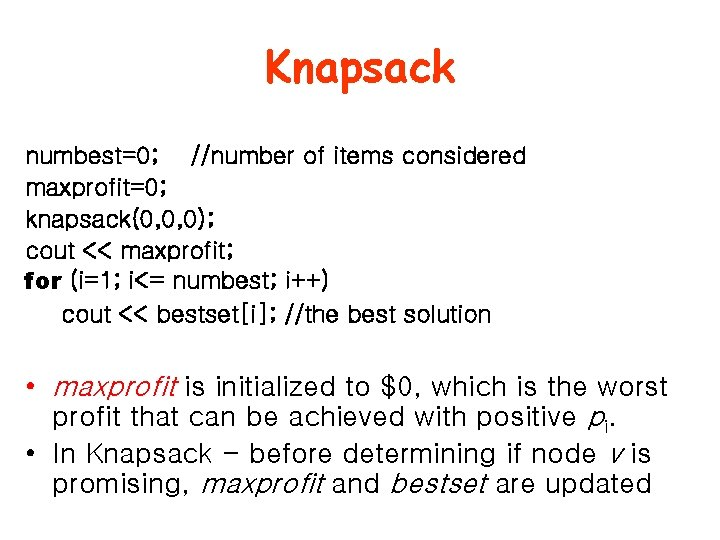Knapsack numbest=0; //number of items considered maxprofit=0; knapsack(0, 0, 0); cout << maxprofit; for (i=1; i<= numbest; i++) cout << bestset[i]; //the best solution • maxprofit is initialized to \$0, which is the worst profit that can be achieved with positive pi. • In Knapsack - before determining if node v is promising, maxprofit and bestset are updated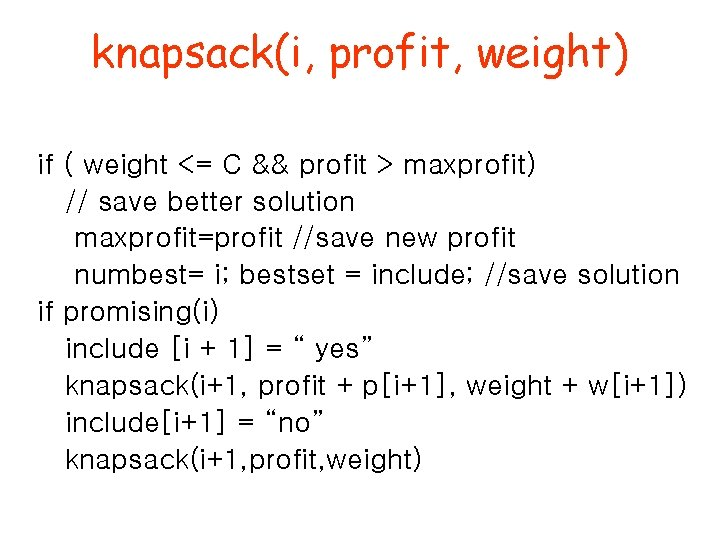knapsack(i, profit, weight) if ( weight <= C && profit > maxprofit) // save better solution maxprofit=profit //save new profit numbest= i; bestset = include; //save solution if promising(i) include [i + 1] = “ yes” knapsack(i+1, profit + p[i+1], weight + w[i+1]) include[i+1] = “no” knapsack(i+1, profit, weight)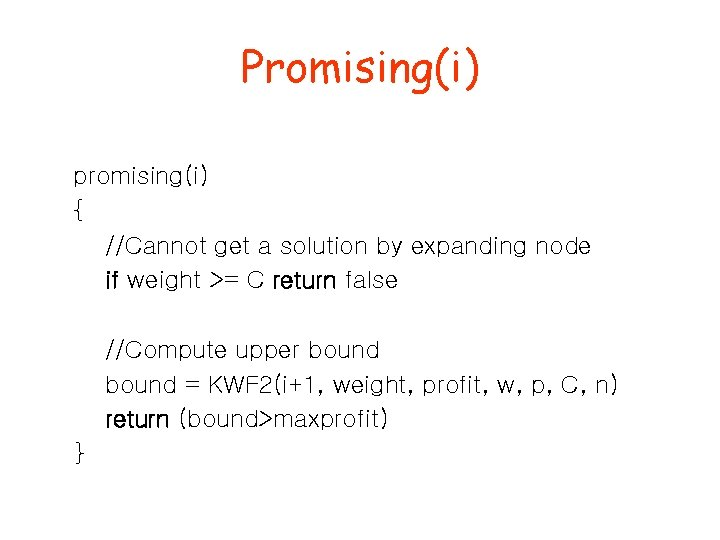Promising(i) promising(i) { //Cannot get a solution by expanding node if weight >= C return false //Compute upper bound = KWF 2(i+1, weight, profit, w, p, C, n) return (bound>maxprofit) }Example • Suppose n = 4, C = 16, and we have the following: i pi 1 \$40 2 \$30 3 \$50 4 \$10 • Note the items by KWF wi pi / wi 2 \$20 5 \$6 10 \$5 5 \$2 are in the correct order needed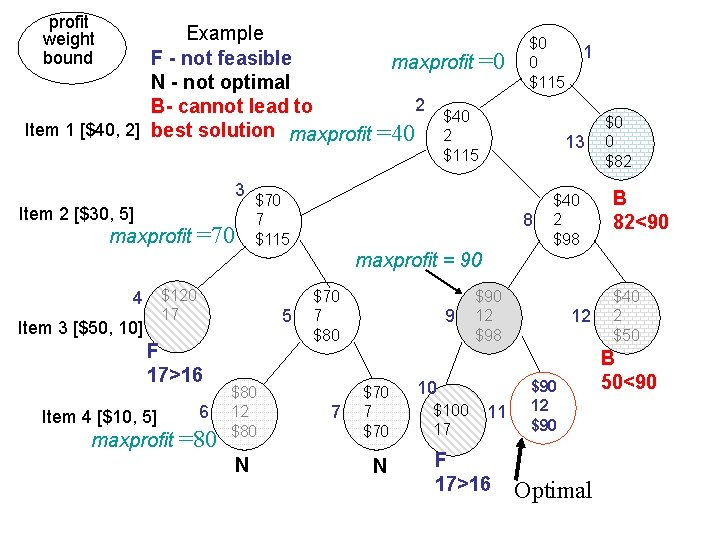profit weight bound Example F - not feasible maxprofit N - not optimal 2 B- cannot lead to \$40 Item 1 [\$40, 2] best solution maxprofit =40 2 =0 \$0 0 \$115 13 \$115 3 Item 2 [\$30, 5] maxprofit =70 1 \$70 7 \$115 8 \$40 2 \$98 \$0 0 \$82 B 82<90 maxprofit = 90 4 \$120 17 Item 3 [\$50, 10] 5 F 17>16 Item 4 [\$10, 5] maxprofit 6 =80 \$80 12 \$80 N \$70 7 \$80 7 9 \$70 7 \$70 N \$90 12 \$98 10 \$100 17 11 F 17>16 12 \$90 Optimal \$40 2 \$50 B 50<90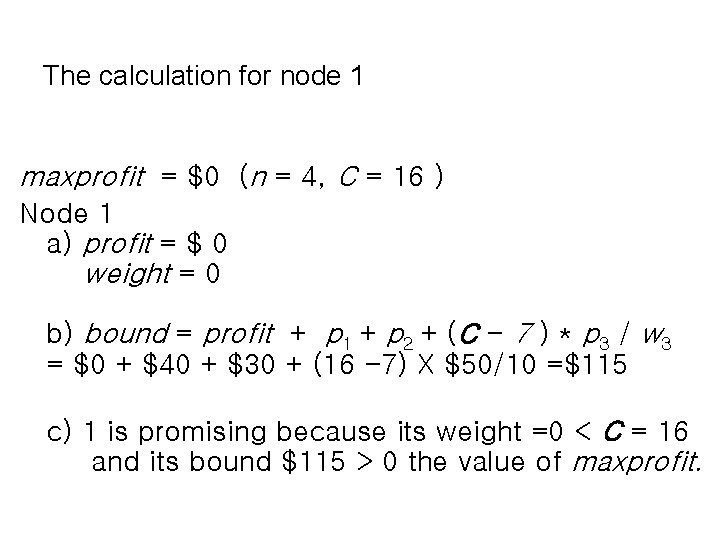The calculation for node 1 maxprofit = \$0 (n = 4, C = 16 ) Node 1 a) profit = \$ 0 weight = 0 b) bound = profit + p 1 + p 2 + (C - 7 ) * p 3 / w 3 = \$0 + \$40 + \$30 + (16 -7) X \$50/10 =\$115 c) 1 is promising because its weight =0 < C = 16 and its bound \$115 > 0 the value of maxprofit.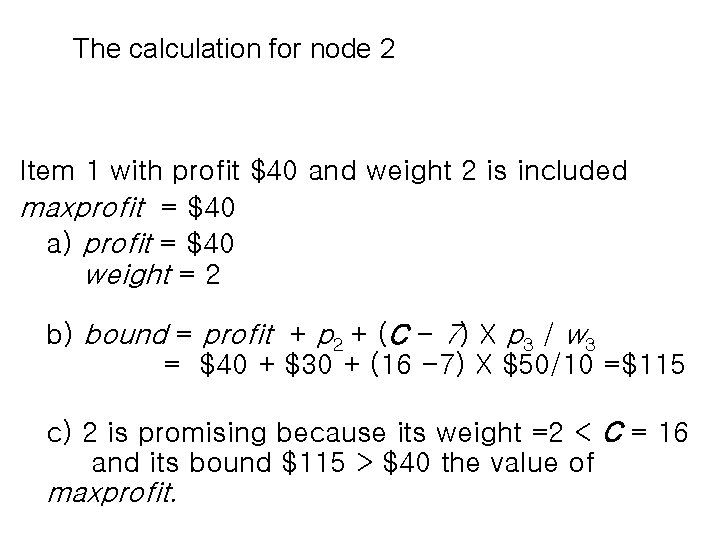The calculation for node 2 Item 1 with profit \$40 and weight 2 is included maxprofit = \$40 a) profit = \$40 weight = 2 b) bound = profit + p 2 + (C - 7) X p 3 / w 3 = \$40 + \$30 + (16 -7) X \$50/10 =\$115 c) 2 is promising because its weight =2 < C = 16 and its bound \$115 > \$40 the value of maxprofit.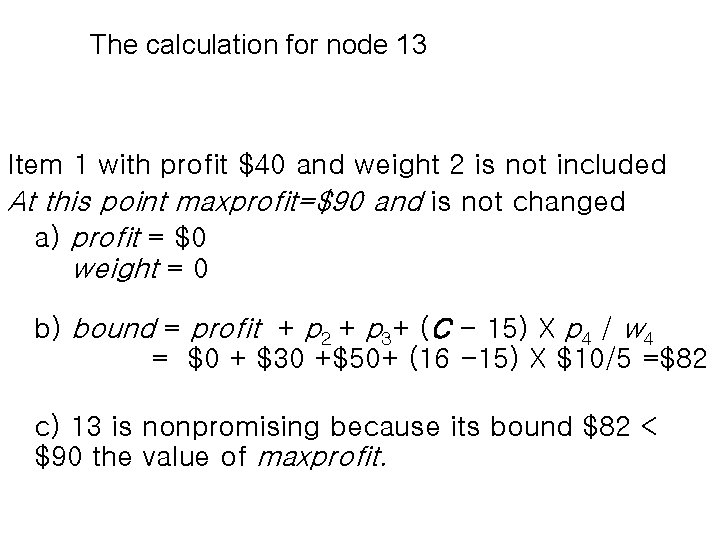The calculation for node 13 Item 1 with profit \$40 and weight 2 is not included At this point maxprofit=\$90 and is not changed a) profit = \$0 weight = 0 b) bound = profit + p 2 + p 3+ (C - 15) X p 4 / w 4 = \$0 + \$30 +\$50+ (16 -15) X \$10/5 =\$82 c) 13 is nonpromising because its bound \$82 < \$90 the value of maxprofit.Worst-case time complexity Check number of nodes: Time complexity: When will it happen? For a given n, W=n Pi = 1, wi=1 (for 1 ≤ i ≤ n-1) Pn=n wn=n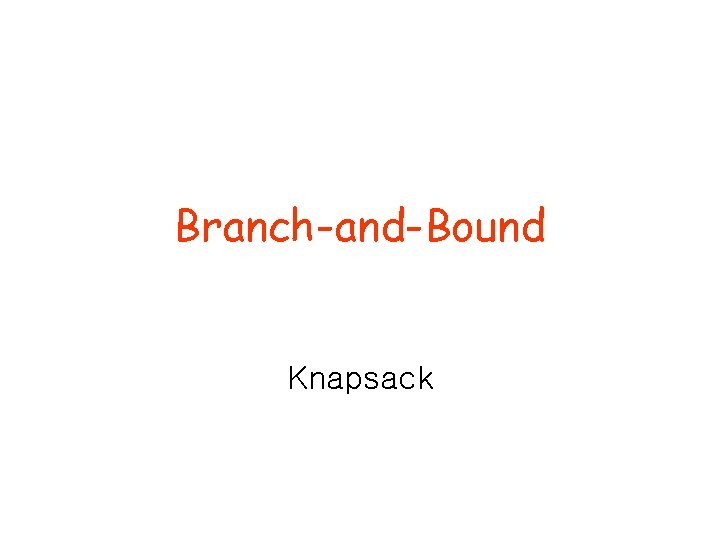Branch-and-Bound Knapsack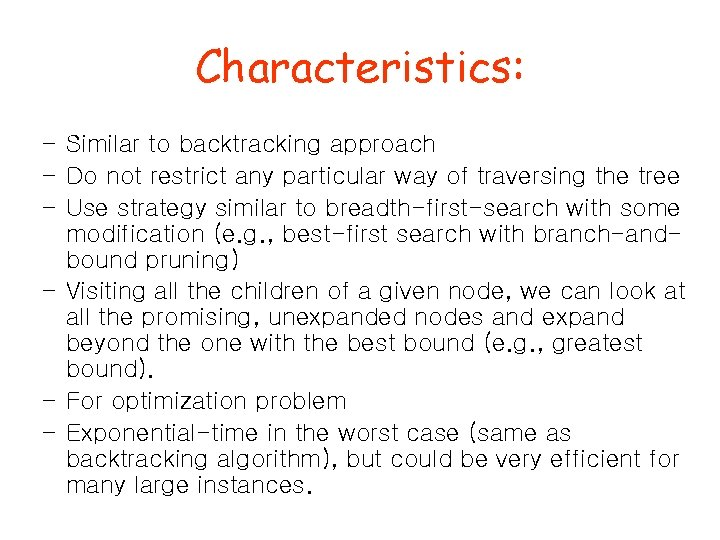Characteristics: – Similar to backtracking approach – Do not restrict any particular way of traversing the tree – Use strategy similar to breadth-first-search with some modification (e. g. , best-first search with branch-andbound pruning) – Visiting all the children of a given node, we can look at all the promising, unexpanded nodes and expand beyond the one with the best bound (e. g. , greatest bound). – For optimization problem – Exponential-time in the worst case (same as backtracking algorithm), but could be very efficient for many large instances.profit weight bound Example F - not feasible maxprofit N - not optimal (1, 1) B- cannot lead to \$40 Item 1 [\$40, 2] best solution maxprofit =40 2 \$115 (2, 1) Item 2 [\$30, 5] maxprofit (3, 1) Item 3 [\$50, 10] =70 =0 \$70 7 \$115 \$0 0 \$115 (0, 0) (2, 2) \$40 2 \$98 \$0 0 \$82 (1, 2) B 82<90 maxprofit = 90 \$120 17 F 17>16 (3, 2) \$70 7 \$80 (3, 3) \$90 12 \$98 (4, 1) Item 4 [\$10, 5] Best-first search with branch-and-bound pruning (Expand the unexplored node with the greatest bound) \$100 17 F 17>16 \$40 2 \$50 \$90 12 \$90 B 50<90 (4, 2) (3, 4)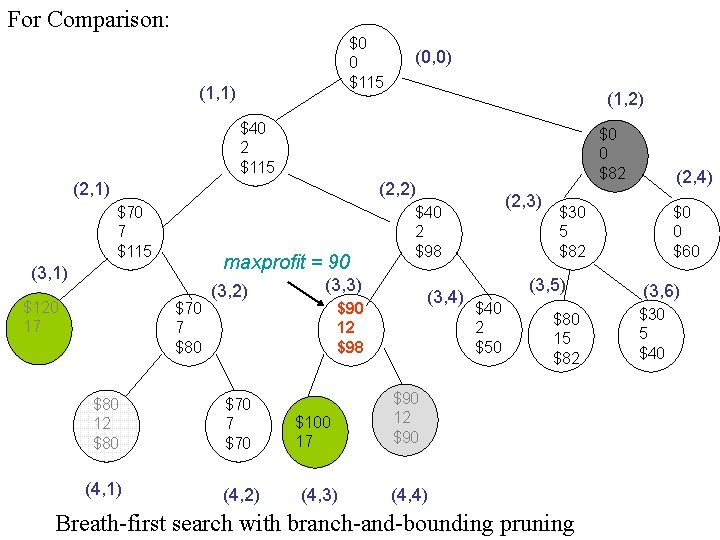For Comparison: \$0 0 \$115 (1, 1) (0, 0) (1, 2) \$40 2 \$115 (2, 1) \$0 0 \$82 (2, 2) \$70 7 \$115 maxprofit = 90 (3, 1) \$120 17 \$70 7 \$80 (3, 2) \$80 12 \$80 \$70 7 \$70 (4, 1) (4, 2) (3, 3) (3, 4) \$90 12 \$98 \$100 17 (4, 3) (2, 3) \$40 2 \$98 \$30 5 \$82 (3, 5) \$40 2 \$50 (2, 4) \$80 15 \$82 \$90 12 \$90 (4, 4) Breath-first search with branch-and-bounding pruning \$0 0 \$60 (3, 6) \$30 5 \$40